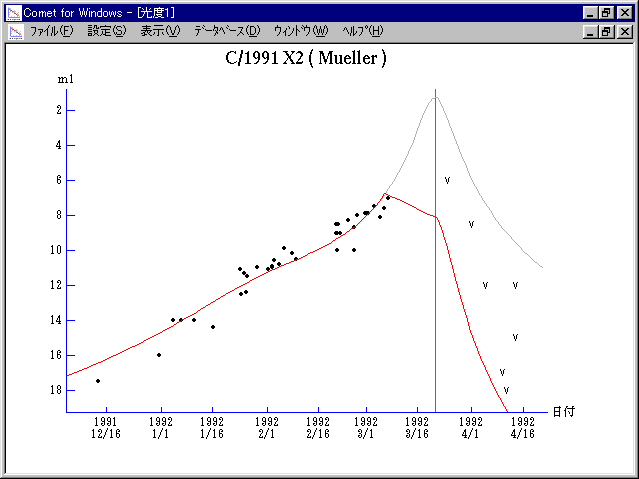# C/1991 X2 ( Mueller )###Profile

 Discovery Date December 13, 1991 Magnitude 17.5 mag Discoverer Jean Mueller (Palomar Sky Survey II)

###Orbital Elements

```     T = 1992 Mar. 21.2096 TT         Peri. = 307.0178
Node  = 288.7876  2000.0
q = 0.198641 AU                  Incl. =  95.5547
```

###Magnitudes Graph

```        m1 = 10.9 + 5 log d + 14.0 log r  [   ,-15]  (             - 1992 Mar.  6)
m1 =  6.5 + 5 log d -  2   log r  [-15,  0]  (1992 Mar.  6 - 1992 Mar. 21)
m1 = 22   + 5 log d + 20   log r  [  0,   ]  (1992 Mar. 21 -             )

* Gray curve is:  m1 = 10.9 + 5 log d + 14.0 log r
```##### The orbital elements are printed on IAUC 5438. The magnitudes graphs are made with Comet for Windows.